Скачать презентацию Scientific Computing with Num Py Sci Py

a27490d85535195f40261a018a147e8d.ppt

• Количество слайдов: 38Scientific Computing with Num. Py & Sci. Py Num. Py Installation and Documentation http: //numpy. scipy. org/ q n Not much on the home page—don’t buy the guide, it’s online (see below) Num. Py page at the Sci. Py site (Better—but not up at the current time) http: //www. scipy. org/Num. Py n The fundamental package for scientific computing with Python. n Contains: q a powerful N-dimensional array object q sophisticated functions that accommodate disparities in array dimensions q tools for integrating C/C++ and Fortran code q linear algebra, Fourier transform, and random number capabilities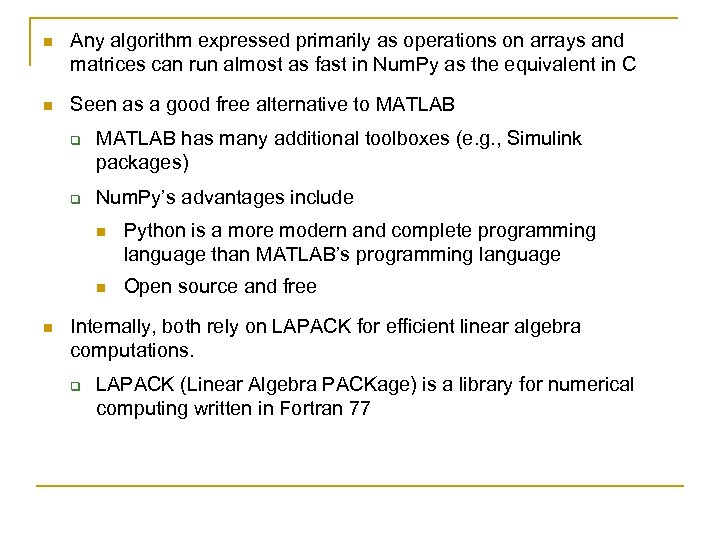n Any algorithm expressed primarily as operations on arrays and matrices can run almost as fast in Num. Py as the equivalent in C n Seen as a good free alternative to MATLAB q q MATLAB has many additional toolboxes (e. g. , Simulink packages) Num. Py’s advantages include n n n Python is a more modern and complete programming language than MATLAB’s programming language Open source and free Internally, both rely on LAPACK for efficient linear algebra computations. q LAPACK (Linear Algebra PACKage) is a library for numerical computing written in Fortran 77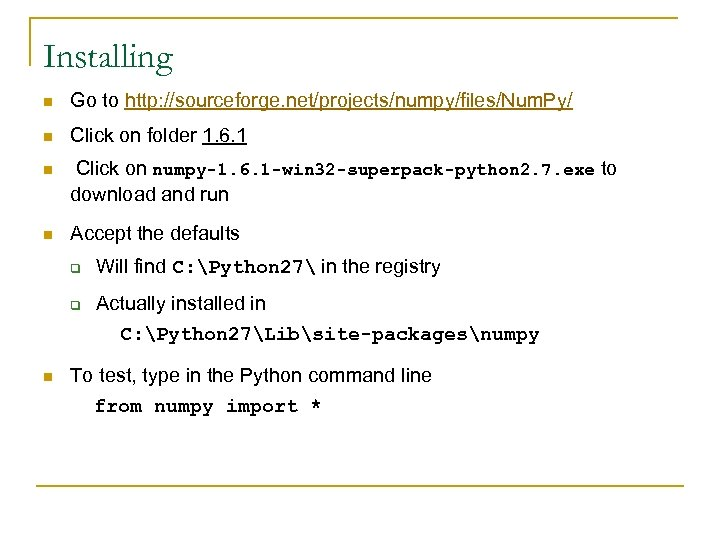Installing n Go to http: //sourceforge. net/projects/numpy/files/Num. Py/ n Click on folder 1. 6. 1 n Click on numpy-1. 6. 1 -win 32 -superpack-python 2. 7. exe to download and run n Accept the defaults q Will find C: Python 27 in the registry q Actually installed in C: Python 27Libsite-packagesnumpy n To test, type in the Python command line from numpy import *Documentation n Num. Py User Guide (work in progress) http: //docs. scipy. org/doc/numpy/user/ q q n Last part documents the C API Like a language reference, not really a tutorial Tentative Num. Py Tutorial (unfinished) http: //www. scipy. org/Tentative_Num. Py_Tutorial q n Good Num. Py Reference Guide (detailed and terse) http: //docs. scipy. org/doc/numpy/reference/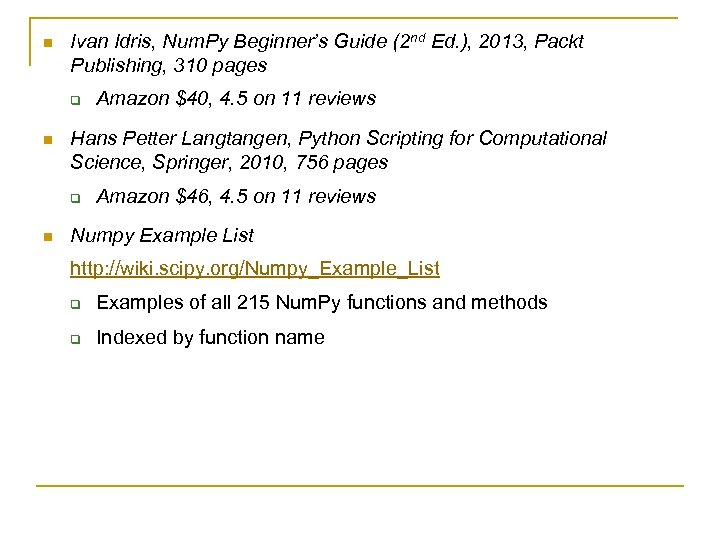n Ivan Idris, Num. Py Beginner’s Guide (2 nd Ed. ), 2013, Packt Publishing, 310 pages q n Hans Petter Langtangen, Python Scripting for Computational Science, Springer, 2010, 756 pages q n Amazon \$40, 4. 5 on 11 reviews Amazon \$46, 4. 5 on 11 reviews Numpy Example List http: //wiki. scipy. org/Numpy_Example_List q Examples of all 215 Num. Py functions and methods q Indexed by function name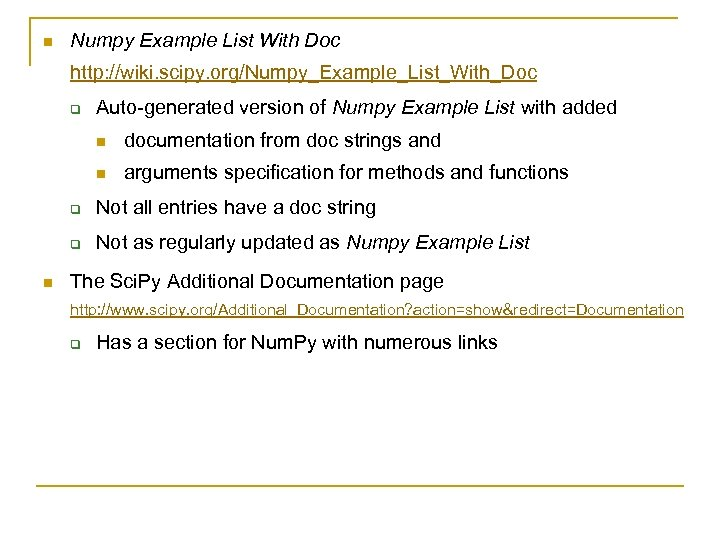n Numpy Example List With Doc http: //wiki. scipy. org/Numpy_Example_List_With_Doc q Auto-generated version of Numpy Example List with added n documentation from doc strings and n arguments specification for methods and functions q q n Not all entries have a doc string Not as regularly updated as Numpy Example List The Sci. Py Additional Documentation page http: //www. scipy. org/Additional_Documentation? action=show&redirect=Documentation q Has a section for Num. Py with numerous links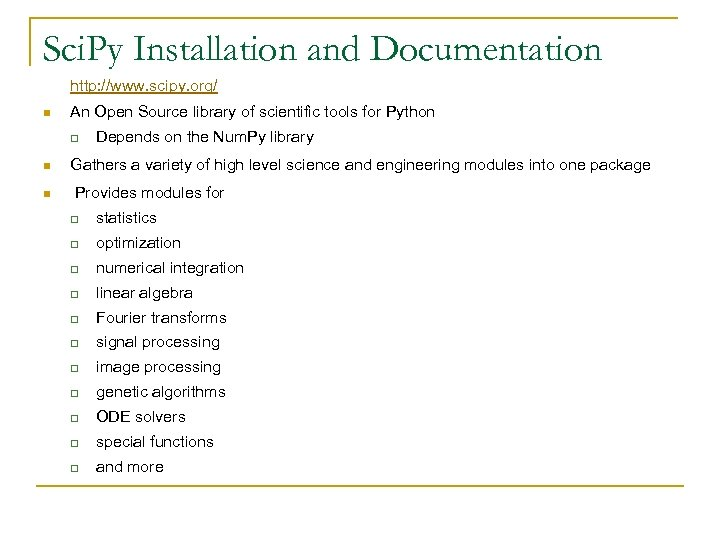Sci. Py Installation and Documentation http: //www. scipy. org/ n An Open Source library of scientific tools for Python q Depends on the Num. Py library n Gathers a variety of high level science and engineering modules into one package n Provides modules for q statistics q optimization q numerical integration q linear algebra q Fourier transforms q signal processing q image processing q genetic algorithms q ODE solvers q special functions q and more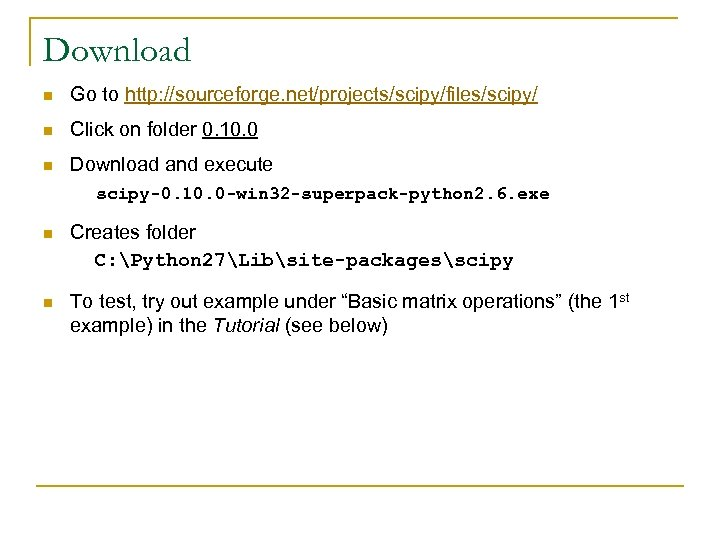Download n Go to http: //sourceforge. net/projects/scipy/files/scipy/ n Click on folder 0. 10. 0 n Download and execute scipy-0. 10. 0 -win 32 -superpack-python 2. 6. exe n Creates folder C: Python 27Libsite-packagesscipy n To test, try out example under “Basic matrix operations” (the 1 st example) in the Tutorial (see below)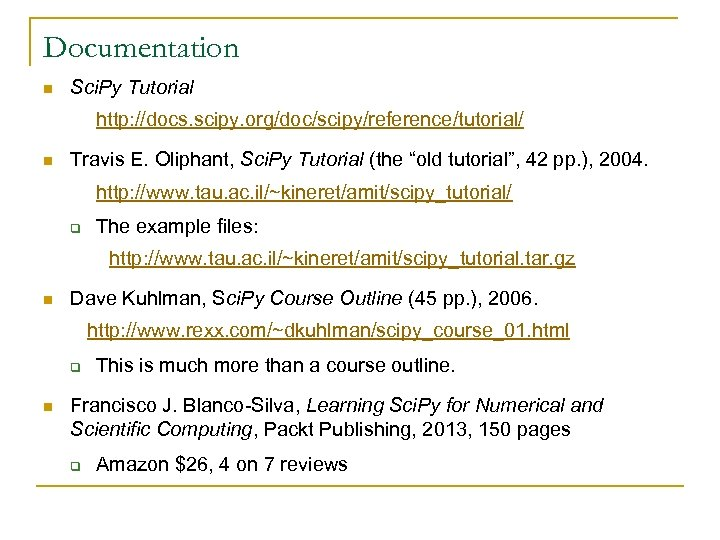Documentation n Sci. Py Tutorial http: //docs. scipy. org/doc/scipy/reference/tutorial/ n Travis E. Oliphant, Sci. Py Tutorial (the “old tutorial”, 42 pp. ), 2004. http: //www. tau. ac. il/~kineret/amit/scipy_tutorial/ q The example files: http: //www. tau. ac. il/~kineret/amit/scipy_tutorial. tar. gz n Dave Kuhlman, Sci. Py Course Outline (45 pp. ), 2006. http: //www. rexx. com/~dkuhlman/scipy_course_01. html q n This is much more than a course outline. Francisco J. Blanco-Silva, Learning Sci. Py for Numerical and Scientific Computing, Packt Publishing, 2013, 150 pages q Amazon \$26, 4 on 7 reviewsn The Sci. Py Community, Sci. Py Reference Guide (Release 0. 12. 0, 1000+ pages), 2013 http: //docs. scipy. org/doc/scipy-ref. pdf q Complete, very much a reference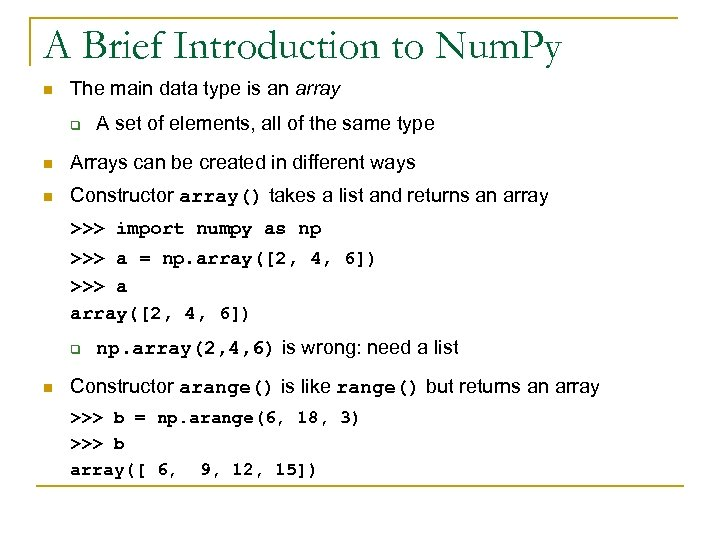A Brief Introduction to Num. Py n The main data type is an array q A set of elements, all of the same type n Arrays can be created in different ways n Constructor array() takes a list and returns an array >>> import numpy as np >>> a = np. array([2, 4, 6]) >>> a array([2, 4, 6]) q n np. array(2, 4, 6) is wrong: need a list Constructor arange() is like range() but returns an array >>> b = np. arange(6, 18, 3) >>> b array([ 6, 9, 12, 15])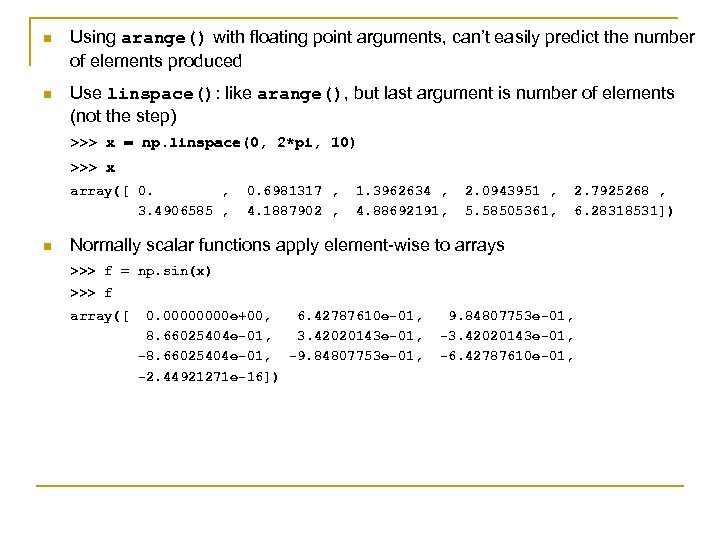n Using arange() with floating point arguments, can’t easily predict the number of elements produced n Use linspace(): like arange(), but last argument is number of elements (not the step) >>> x = np. linspace(0, 2*pi, 10) >>> x array([ 0. , 3. 4906585 , n 0. 6981317 , 4. 1887902 , 1. 3962634 , 4. 88692191, 2. 0943951 , 5. 58505361, 2. 7925268 , 6. 28318531]) Normally scalar functions apply element-wise to arrays >>> f = np. sin(x) >>> f array([ 0. 0000 e+00, 6. 42787610 e-01, 8. 66025404 e-01, 3. 42020143 e-01, -8. 66025404 e-01, -9. 84807753 e-01, -2. 44921271 e-16]) 9. 84807753 e-01, -3. 42020143 e-01, -6. 42787610 e-01,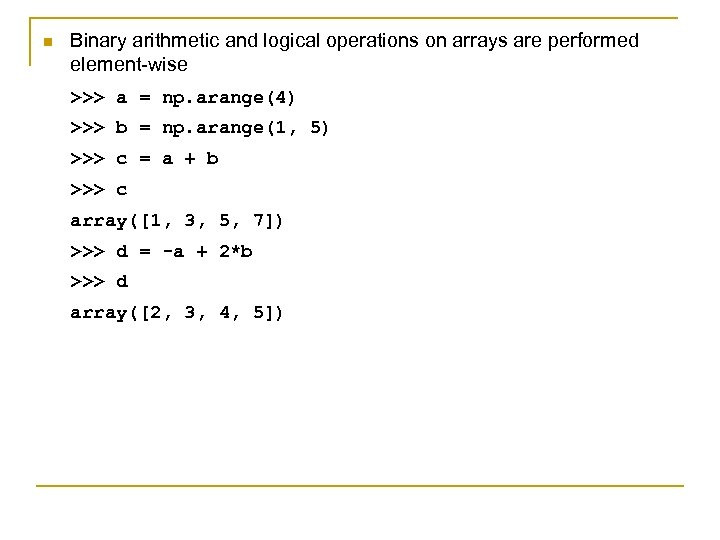n Binary arithmetic and logical operations on arrays are performed element-wise >>> a = np. arange(4) >>> b = np. arange(1, 5) >>> c = a + b >>> c array([1, 3, 5, 7]) >>> d = -a + 2*b >>> d array([2, 3, 4, 5])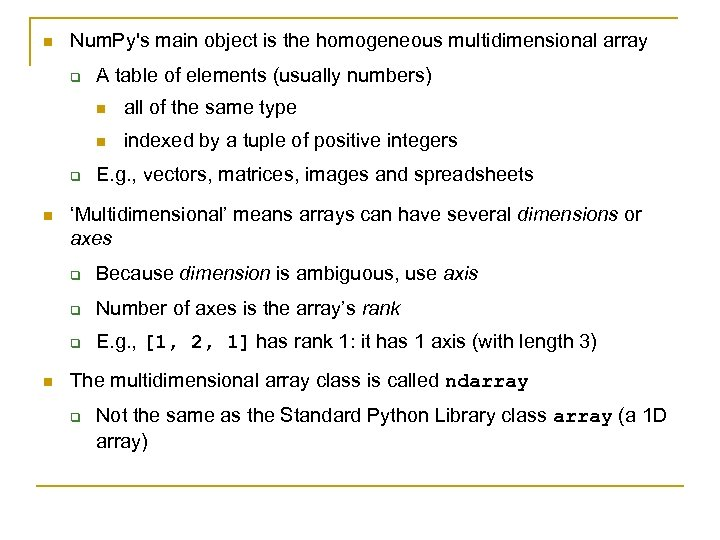n Num. Py's main object is the homogeneous multidimensional array q A table of elements (usually numbers) n n q n all of the same type indexed by a tuple of positive integers E. g. , vectors, matrices, images and spreadsheets ‘Multidimensional’ means arrays can have several dimensions or axes q q Number of axes is the array’s rank q n Because dimension is ambiguous, use axis E. g. , [1, 2, 1] has rank 1: it has 1 axis (with length 3) The multidimensional array class is called ndarray q Not the same as the Standard Python Library class array (a 1 D array)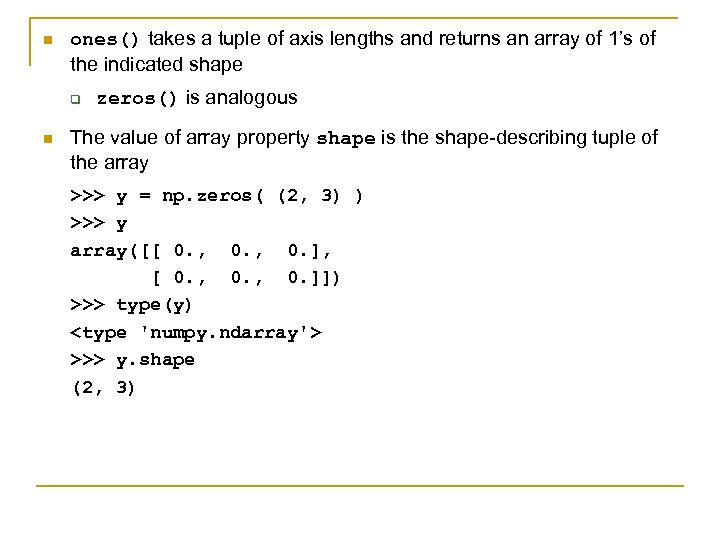n ones() takes a tuple of axis lengths and returns an array of 1’s of the indicated shape q n zeros() is analogous The value of array property shape is the shape-describing tuple of the array >>> y = np. zeros( (2, 3) ) >>> y array([[ 0. , 0. ], [ 0. , 0. ]]) >>> type(y) >>> y. shape (2, 3)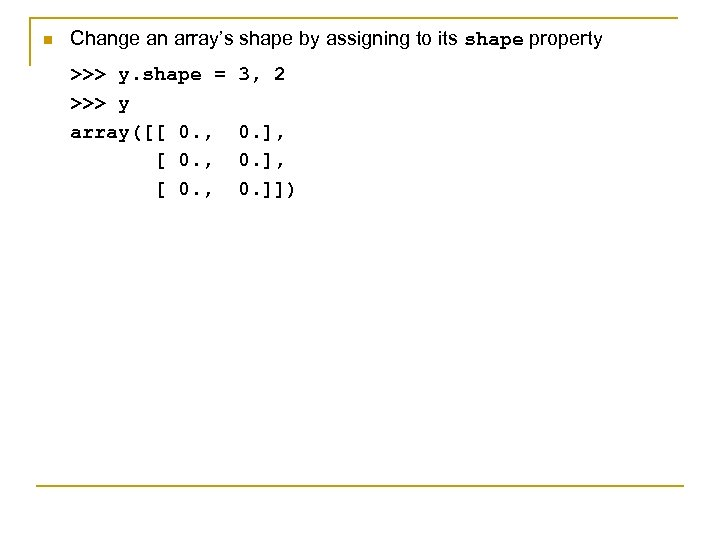n Change an array’s shape by assigning to its shape property >>> y. shape = >>> y array([[ 0. , 3, 2 0. ], 0. ]])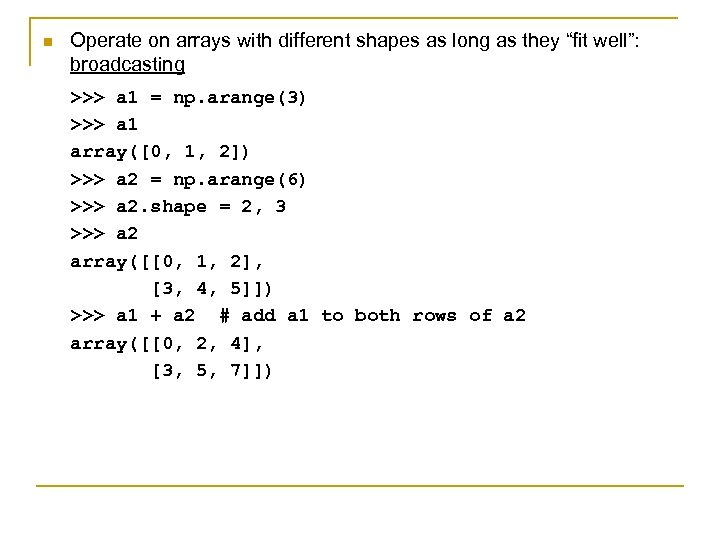n Operate on arrays with different shapes as long as they “fit well”: broadcasting >>> a 1 = np. arange(3) >>> a 1 array([0, 1, 2]) >>> a 2 = np. arange(6) >>> a 2. shape = 2, 3 >>> a 2 array([[0, 1, 2], [3, 4, 5]]) >>> a 1 + a 2 # add a 1 to both rows of a 2 array([[0, 2, 4], [3, 5, 7]])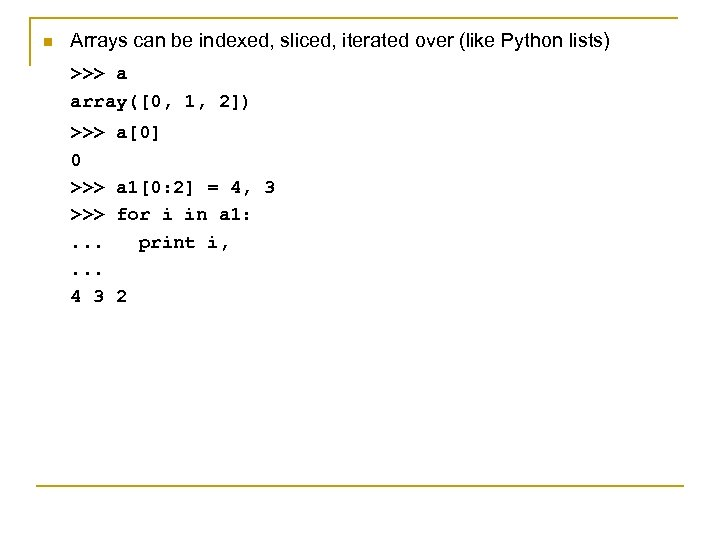n Arrays can be indexed, sliced, iterated over (like Python lists) >>> a array([0, 1, 2]) >>> 0 >>>. . . 4 3 a a 1[0: 2] = 4, 3 for i in a 1: print i, 2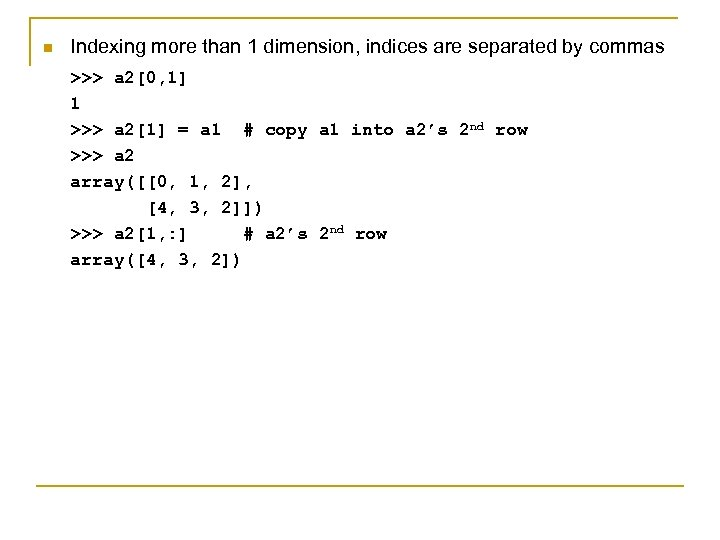n Indexing more than 1 dimension, indices are separated by commas >>> a 2[0, 1] 1 >>> a 2 = a 1 # copy a 1 into a 2’s 2 nd row >>> a 2 array([[0, 1, 2], [4, 3, 2]]) >>> a 2[1, : ] # a 2’s 2 nd row array([4, 3, 2])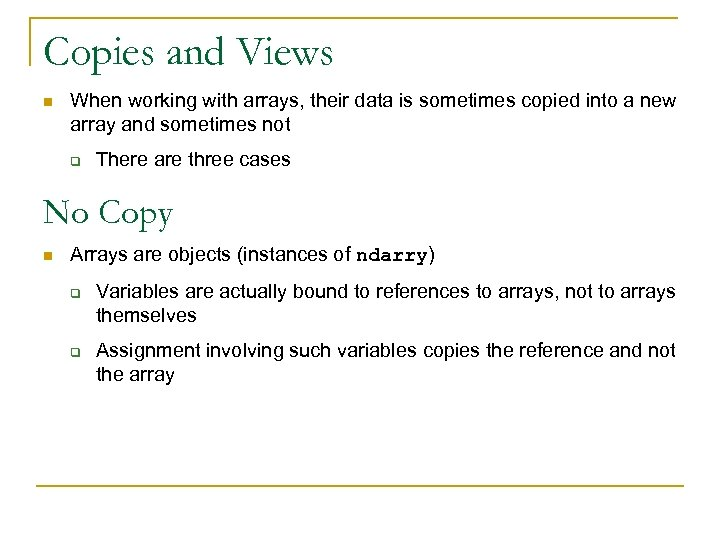Copies and Views n When working with arrays, their data is sometimes copied into a new array and sometimes not q There are three cases No Copy n Arrays are objects (instances of ndarry) q q Variables are actually bound to references to arrays, not to arrays themselves Assignment involving such variables copies the reference and not the array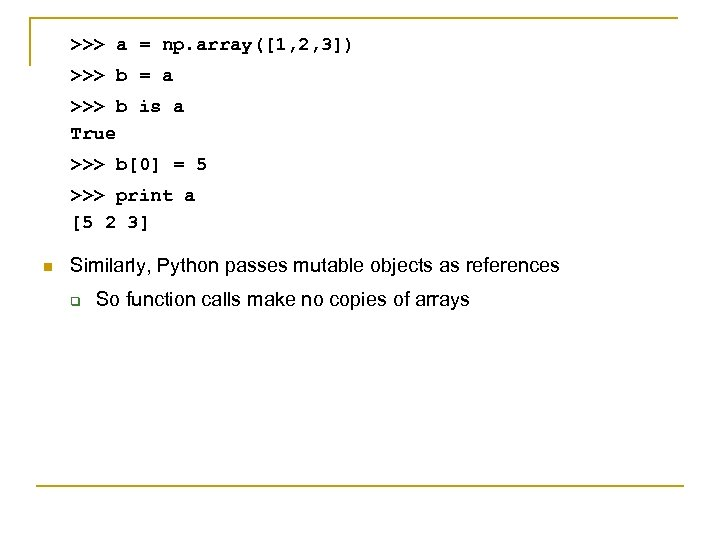>>> a = np. array([1, 2, 3]) >>> b = a >>> b is a True >>> b = 5 >>> print a [5 2 3] n Similarly, Python passes mutable objects as references q So function calls make no copies of arrays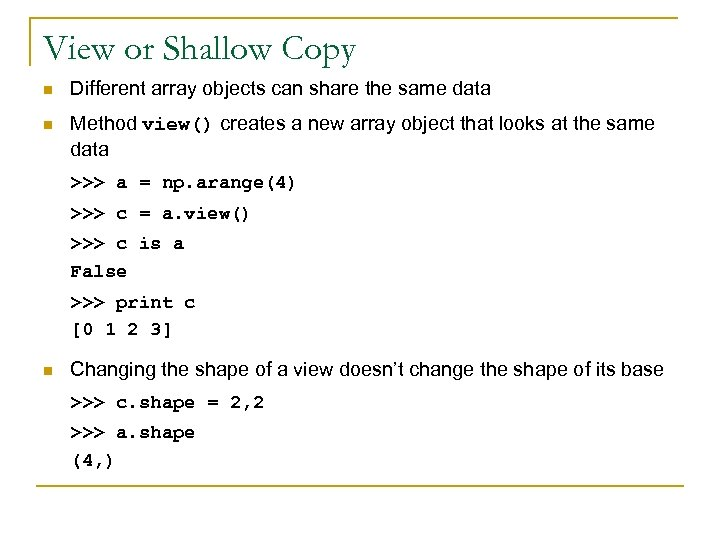View or Shallow Copy n Different array objects can share the same data n Method view() creates a new array object that looks at the same data >>> a = np. arange(4) >>> c = a. view() >>> c is a False >>> print c [0 1 2 3] n Changing the shape of a view doesn’t change the shape of its base >>> c. shape = 2, 2 >>> a. shape (4, )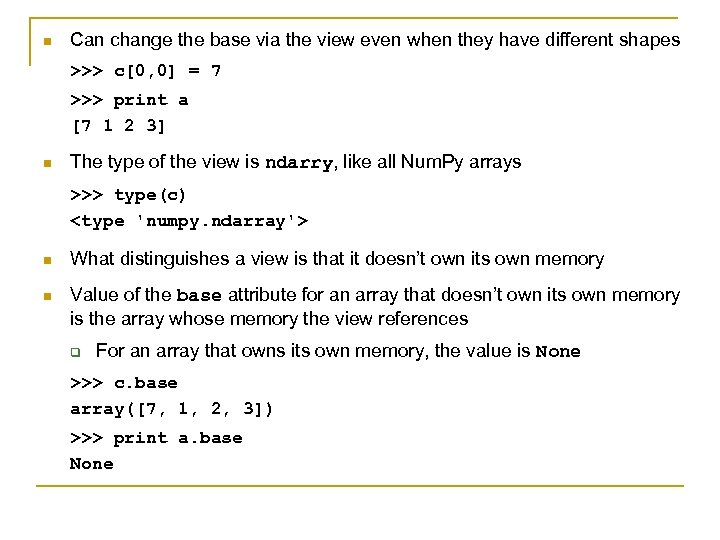n Can change the base via the view even when they have different shapes >>> c[0, 0] = 7 >>> print a [7 1 2 3] n The type of the view is ndarry, like all Num. Py arrays >>> type(c) n What distinguishes a view is that it doesn’t own its own memory n Value of the base attribute for an array that doesn’t own its own memory is the array whose memory the view references q For an array that owns its own memory, the value is None >>> c. base array([7, 1, 2, 3]) >>> print a. base None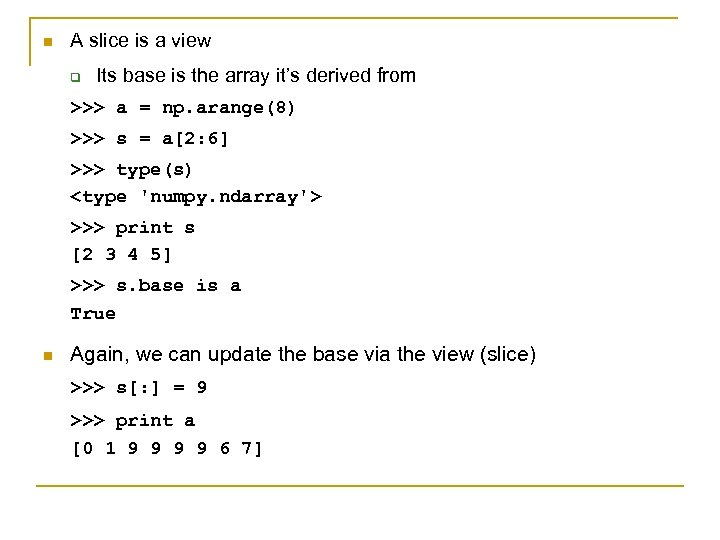n A slice is a view q Its base is the array it’s derived from >>> a = np. arange(8) >>> s = a[2: 6] >>> type(s) >>> print s [2 3 4 5] >>> s. base is a True n Again, we can update the base via the view (slice) >>> s[: ] = 9 >>> print a [0 1 9 9 6 7]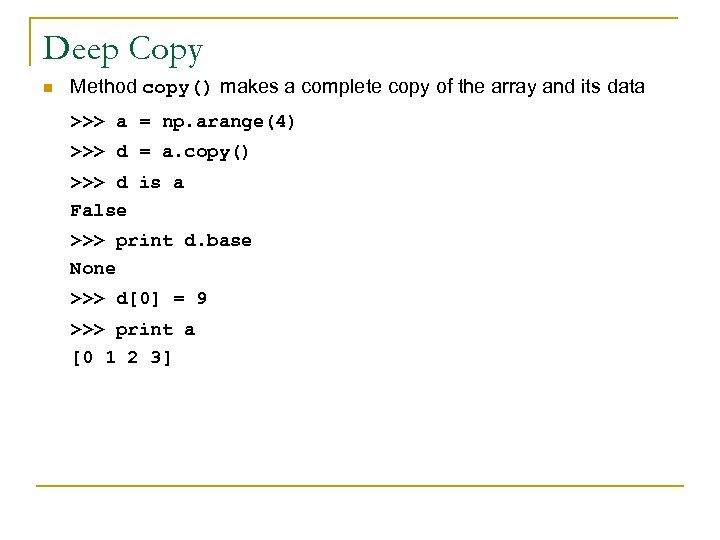Deep Copy n Method copy() makes a complete copy of the array and its data >>> a = np. arange(4) >>> d = a. copy() >>> d is a False >>> print d. base None >>> d = 9 >>> print a [0 1 2 3]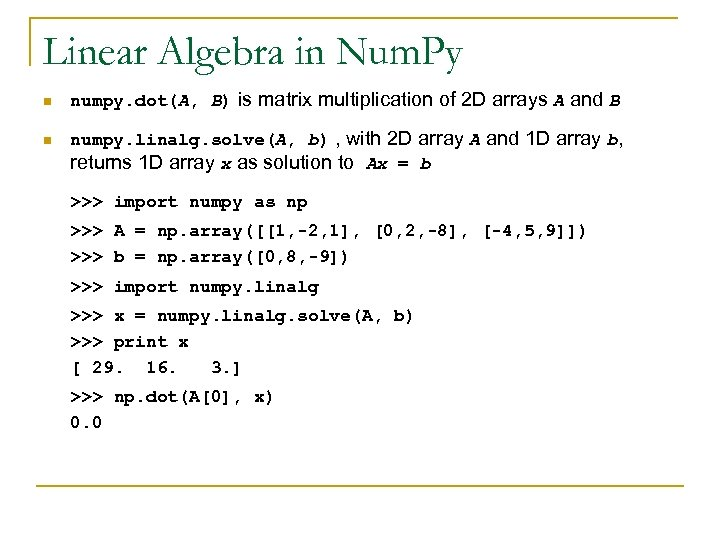Linear Algebra in Num. Py n n numpy. dot(A, B) is matrix multiplication of 2 D arrays A and B numpy. linalg. solve(A, b) , with 2 D array A and 1 D array b, returns 1 D array x as solution to Ax = b >>> import numpy as np >>> A = np. array([[1, -2, 1], [0, 2, -8], [-4, 5, 9]]) >>> b = np. array([0, 8, -9]) >>> import numpy. linalg >>> x = numpy. linalg. solve(A, b) >>> print x [ 29. 16. 3. ] >>> np. dot(A, x) 0. 0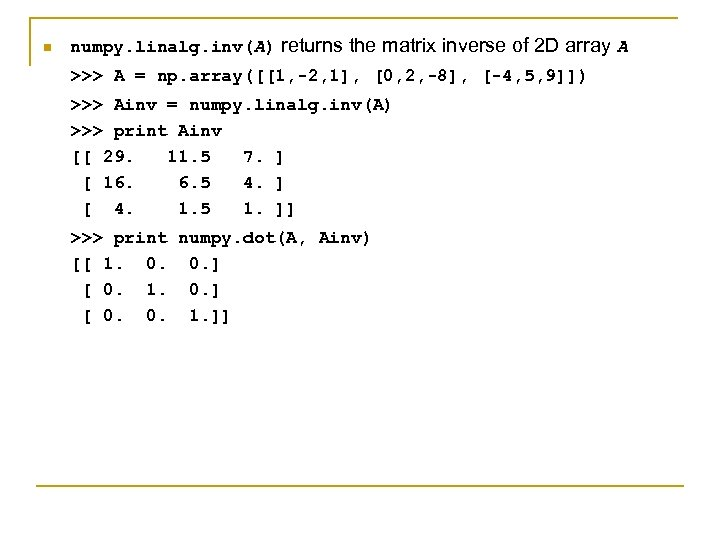n numpy. linalg. inv(A) returns the matrix inverse of 2 D array A >>> A = np. array([[1, -2, 1], [0, 2, -8], [-4, 5, 9]]) >>> Ainv = numpy. linalg. inv(A) >>> print Ainv [[ 29. 11. 5 7. ] [ 16. 6. 5 4. ] [ 4. 1. 5 1. ]] >>> print numpy. dot(A, Ainv) [[ 1. 0. 0. ] [ 0. 1. 0. ] [ 0. 0. 1. ]]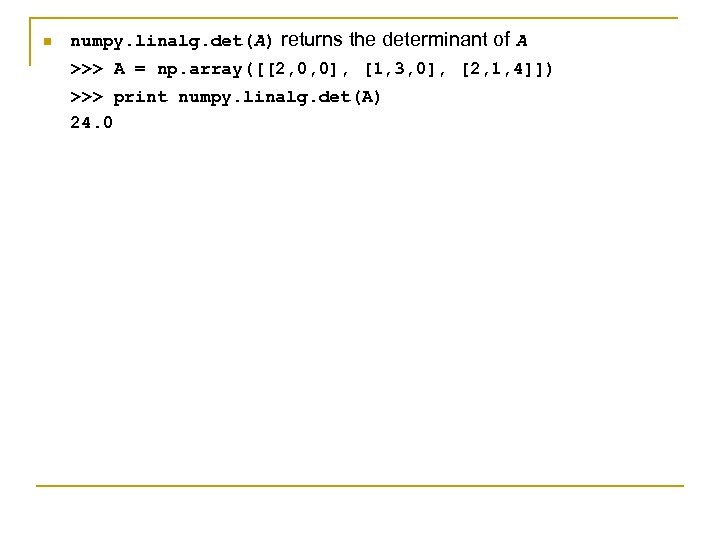n numpy. linalg. det(A) returns the determinant of A >>> A = np. array([[2, 0, 0], [1, 3, 0], [2, 1, 4]]) >>> print numpy. linalg. det(A) 24. 0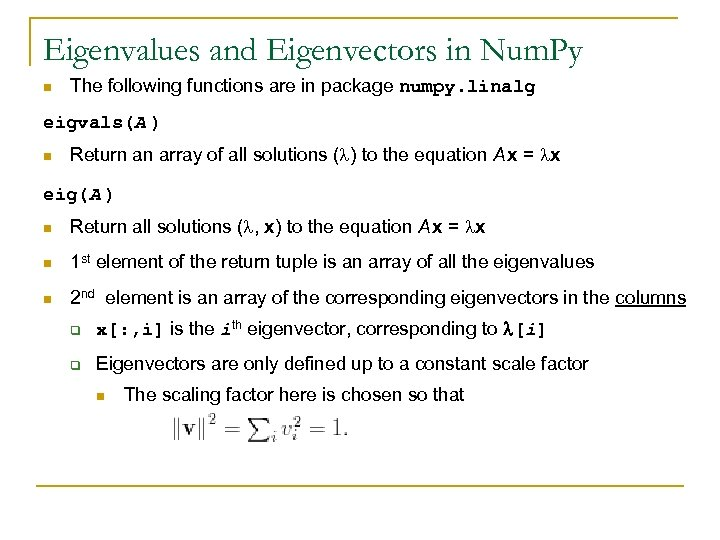Eigenvalues and Eigenvectors in Num. Py n The following functions are in package numpy. linalg eigvals(A ) n Return an array of all solutions ( ) to the equation A x = x eig(A ) n Return all solutions ( , x) to the equation A x = x n 1 st element of the return tuple is an array of all the eigenvalues n 2 nd element is an array of the corresponding eigenvectors in the columns q x[: , i] is the ith eigenvector, corresponding to [i] q Eigenvectors are only defined up to a constant scale factor n The scaling factor here is chosen so that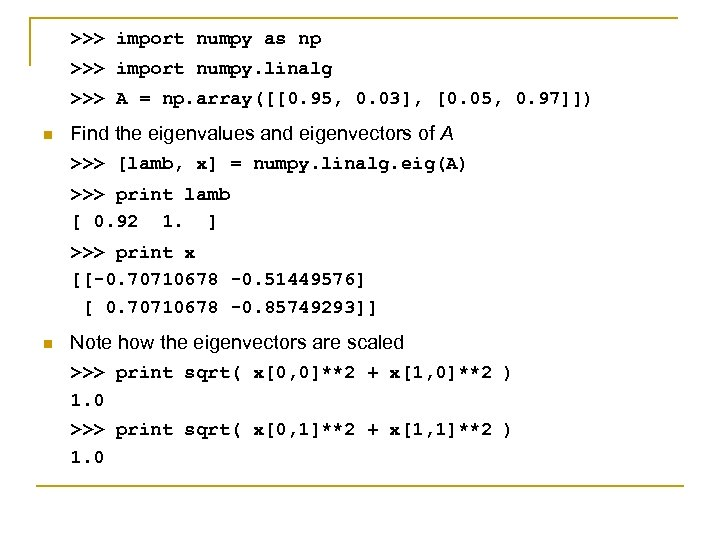>>> import numpy as np >>> import numpy. linalg >>> A = np. array([[0. 95, 0. 03], [0. 05, 0. 97]]) n Find the eigenvalues and eigenvectors of A >>> [lamb, x] = numpy. linalg. eig(A) >>> print lamb [ 0. 92 1. ] >>> print x [[-0. 70710678 -0. 51449576] [ 0. 70710678 -0. 85749293]] n Note how the eigenvectors are scaled >>> print sqrt( x[0, 0]**2 + x[1, 0]**2 ) 1. 0 >>> print sqrt( x[0, 1]**2 + x[1, 1]**2 ) 1. 0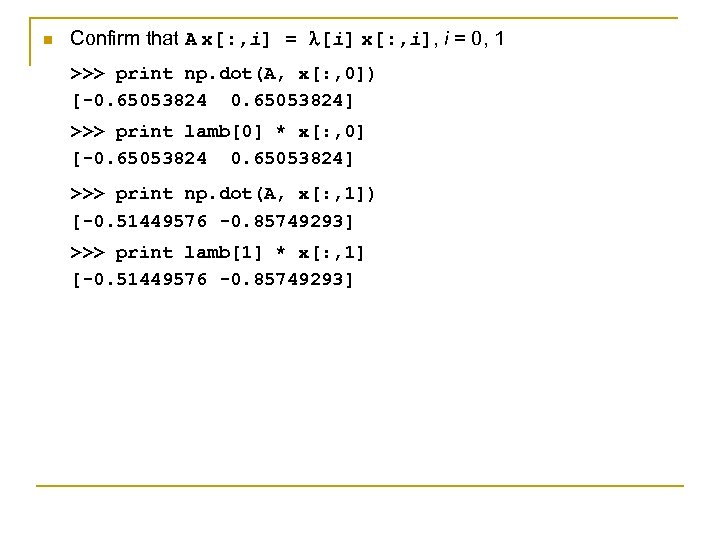n Confirm that A x[: , i] = [i] x[: , i], i = 0, 1 >>> print np. dot(A, x[: , 0]) [-0. 65053824] >>> print lamb * x[: , 0] [-0. 65053824] >>> print np. dot(A, x[: , 1]) [-0. 51449576 -0. 85749293] >>> print lamb * x[: , 1] [-0. 51449576 -0. 85749293]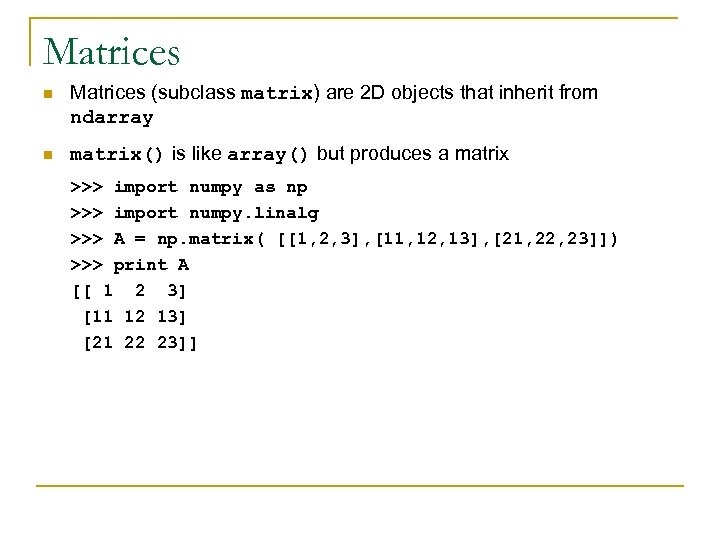Matrices n Matrices (subclass matrix) are 2 D objects that inherit from ndarray n matrix() is like array() but produces a matrix >>> import numpy as np >>> import numpy. linalg >>> A = np. matrix( [[1, 2, 3], [11, 12, 13], [21, 22, 23]]) >>> print A [[ 1 2 3] [11 12 13] [21 22 23]]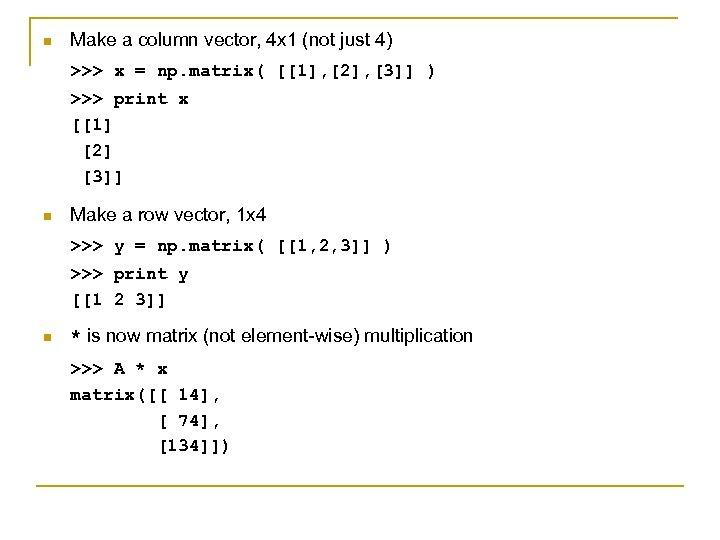n Make a column vector, 4 x 1 (not just 4) >>> x = np. matrix( [, , ] ) >>> print x [  ] n Make a row vector, 1 x 4 >>> y = np. matrix( [[1, 2, 3]] ) >>> print y [[1 2 3]] n * is now matrix (not element-wise) multiplication >>> A * x matrix([[ 14], [ 74], ])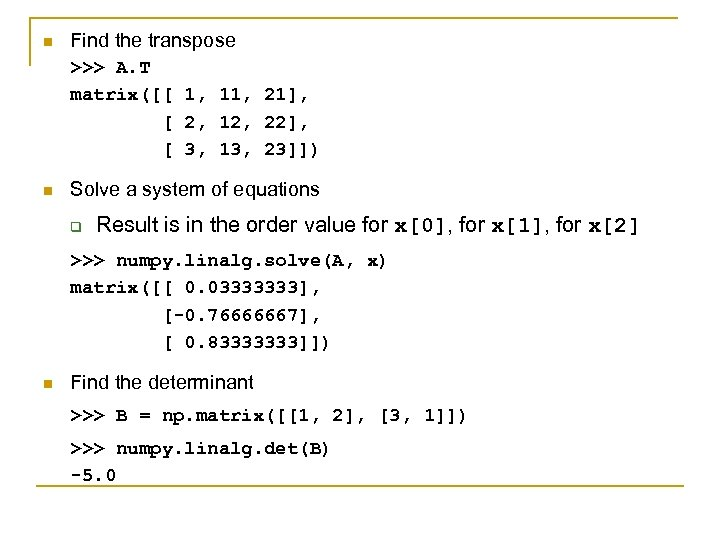n Find the transpose >>> A. T matrix([[ 1, 11, 21], [ 2, 12, 22], [ 3, 13, 23]]) n Solve a system of equations q Result is in the order value for x, for x, for x >>> numpy. linalg. solve(A, x) matrix([[ 0. 03333333], [-0. 76666667], [ 0. 83333333]]) n Find the determinant >>> B = np. matrix([[1, 2], [3, 1]]) >>> numpy. linalg. det(B) -5. 0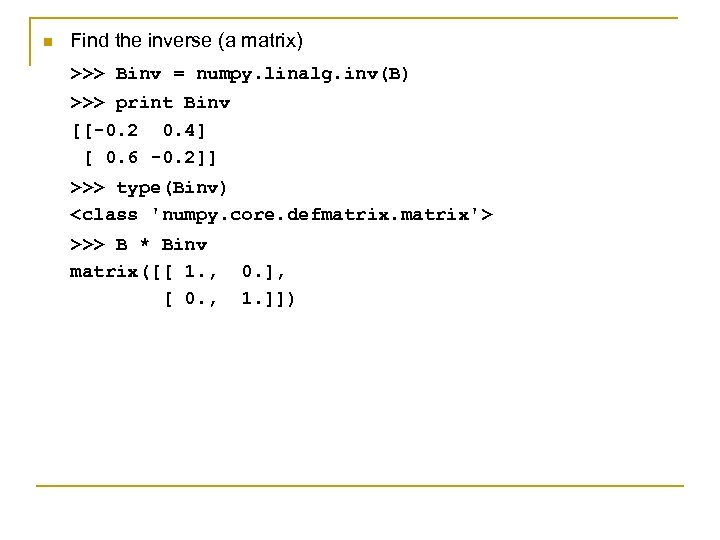n Find the inverse (a matrix) >>> Binv = numpy. linalg. inv(B) >>> print Binv [[-0. 2 0. 4] [ 0. 6 -0. 2]] >>> type(Binv) >>> B * Binv matrix([[ 1. , [ 0. , 0. ], 1. ]])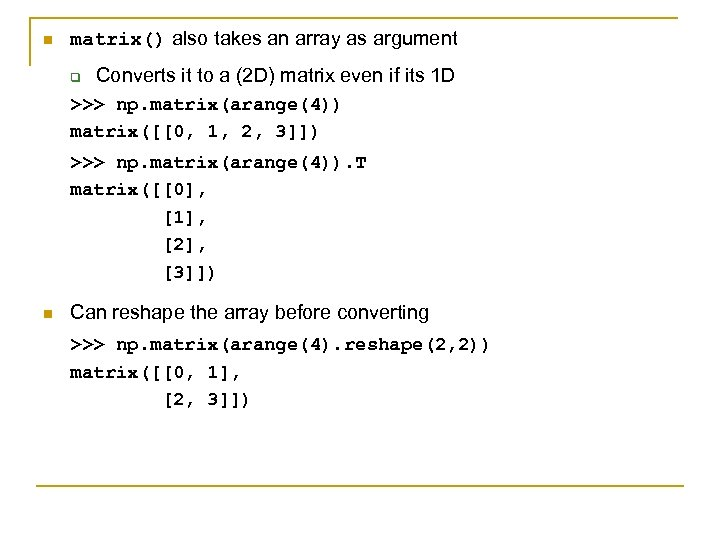n matrix() also takes an array as argument q Converts it to a (2 D) matrix even if its 1 D >>> np. matrix(arange(4)) matrix([[0, 1, 2, 3]]) >>> np. matrix(arange(4)). T matrix([, , , ]) n Can reshape the array before converting >>> np. matrix(arange(4). reshape(2, 2)) matrix([[0, 1], [2, 3]])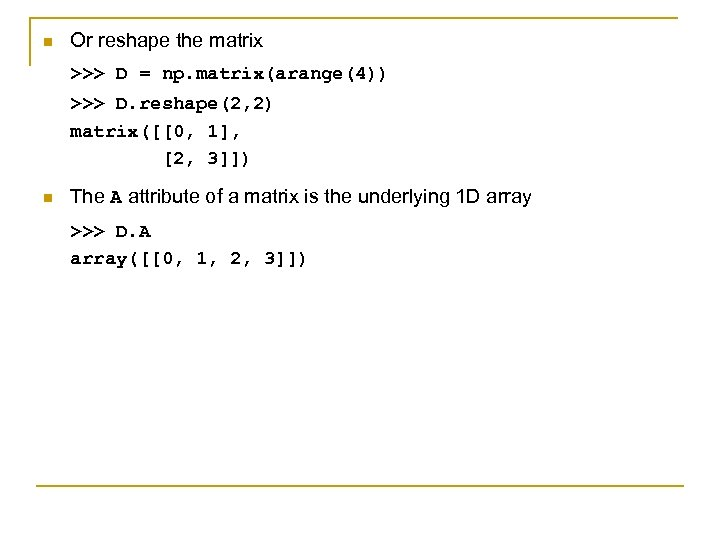n Or reshape the matrix >>> D = np. matrix(arange(4)) >>> D. reshape(2, 2) matrix([[0, 1], [2, 3]]) n The A attribute of a matrix is the underlying 1 D array >>> D. A array([[0, 1, 2, 3]])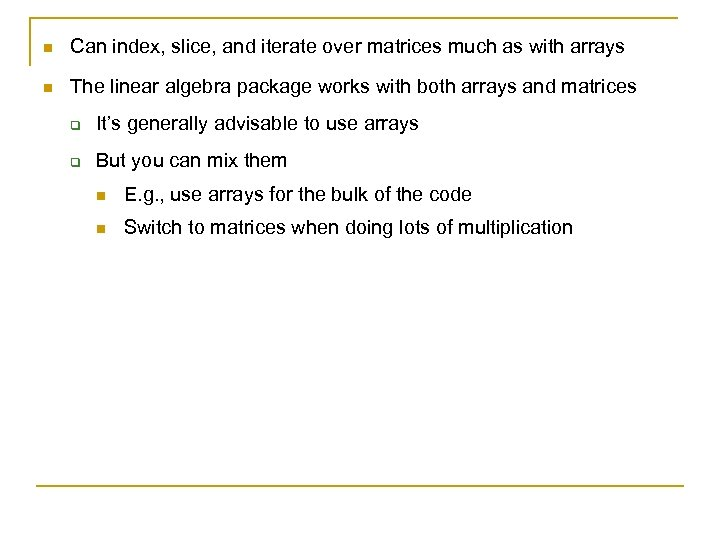n Can index, slice, and iterate over matrices much as with arrays n The linear algebra package works with both arrays and matrices q It’s generally advisable to use arrays q But you can mix them n E. g. , use arrays for the bulk of the code n Switch to matrices when doing lots of multiplication